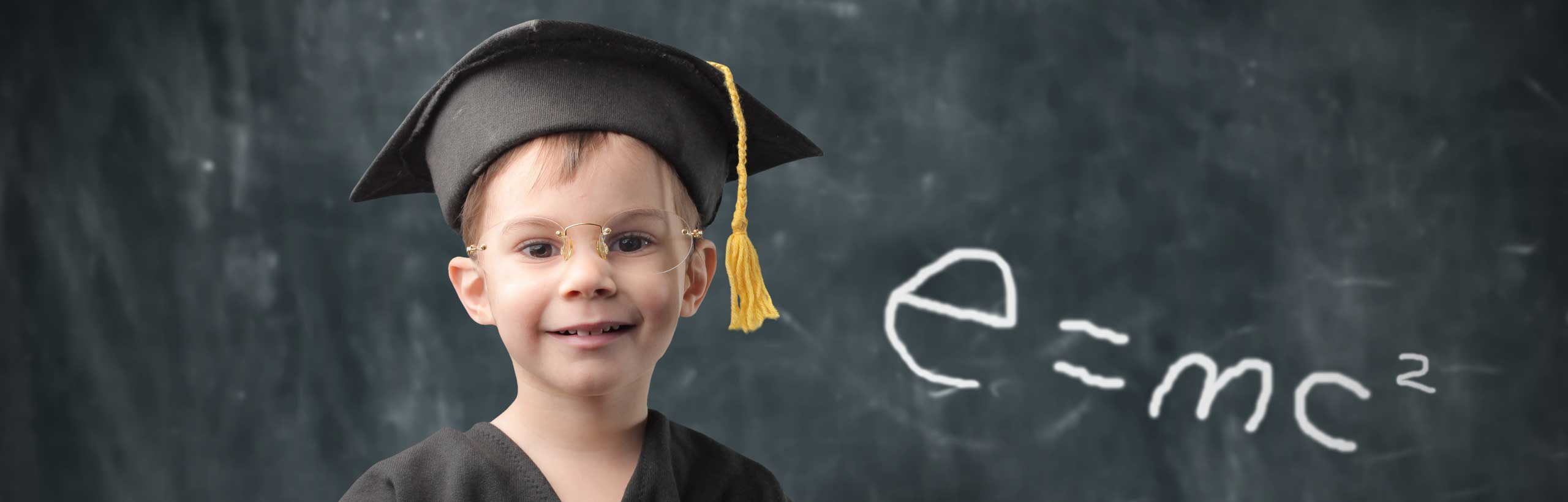# Prime Factorization of Monomials - Questions With Detailed Solutions

What is prime factorization of monomials? Grade 11 examples and questions are presented along with Solutions and explanations included.

## Review of Monomials and Prime Factorization

A monomial is the product of a real number and variables raised to non negative integer powers.
Example of monomials: 2 x , -5 x 2y , 30 x 2y 4
What is prime factorization of monomials?
The prime factorization of a monomials is obtained by writing the whole number in the monomial in prime factorization followed by the product of the variables.
Examples of monomials in prime factorization form
1) 10 x = 2 � 5 � x
2) 20 x 2 = 2 � 2 � 5 � xx
3) - 30 x 2y 3 = - 2 � 3 � 5 � xx � y � yy

## Questions

1. Which of the following is not a prime factorization?
a)2 � 10 � x
b) 2 � 7 � xx
c) 4 � 4 � 4 � xxyyy
d) 2 � 2 � 2 � 3 � xxyy
2. What is the prime factorization of the following monomials?
a) 28 x y 2
b) 32 x 3y
c) 70 x 3y 3
d) 120 x 2y 2
3. Find the prime factorizations of 5 x y 2 and 20 x 3y and then the prime factorization of 100 x 4y 3 knowing that 100 x 4y 3 = (5 x y 2) � (20 x 3y)

## Solutions to the Above Questions

1. Solution
a) The 10 in 2 � 10 � x is not a prime number and therefore 2 � 10 � x is not a prime factorization.
b) 2 � 7 � xx is a prime factorization.
c) The 4 in 4 � 4 � 4 � xxy is not a prime number and therefore 4 � 4 � 4 � xxy is not a prime factorization.
d) 2 � 2 � 2 � 3 � xxyy is a prime factorization.

2. Solution
a) 28 x y 2 = 2 � 2 � 7 � x � y � y
b) 32 x 3y = 2 � 2 � 2 � 2 � 2 � x � x � x � y
c) 70 x 3y 3 = 2 �5 � 7 � x � x � x � y � y � y
d) 120 x 2y 2 = 2 � 2 � 2 � 3 � 5 � x� x � y

3. Solution
The prime factorization of 5 x y 2 is.
5 x y 2 = 5 � x � y � y
The prime factorization of 20 x 3y is.
20 x 3y = 2 � 2 � 5 � x � x � x � y
The prime factorization of 100 x 4y 3 is.
100 x 4y 3 = (5 x y 2) � (20 x 3y) =
(5 � x � y � y ) � (2 � 2 � 5 � x � x � x � y ) =
2 � 2 � 5 � 5 x � x � x � x � y � y � y